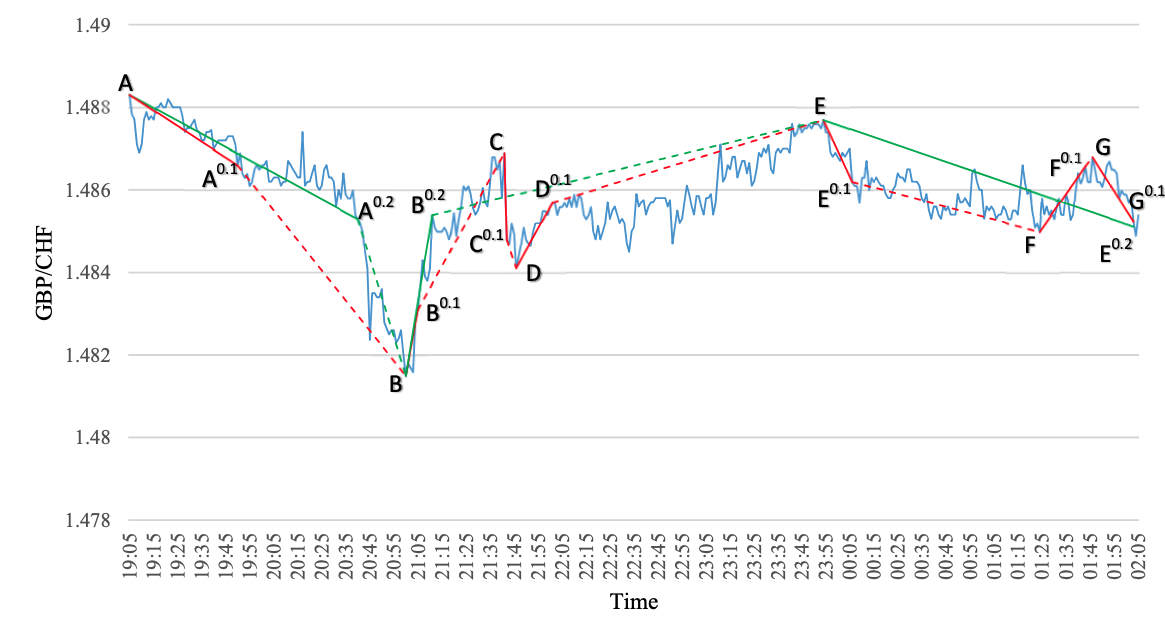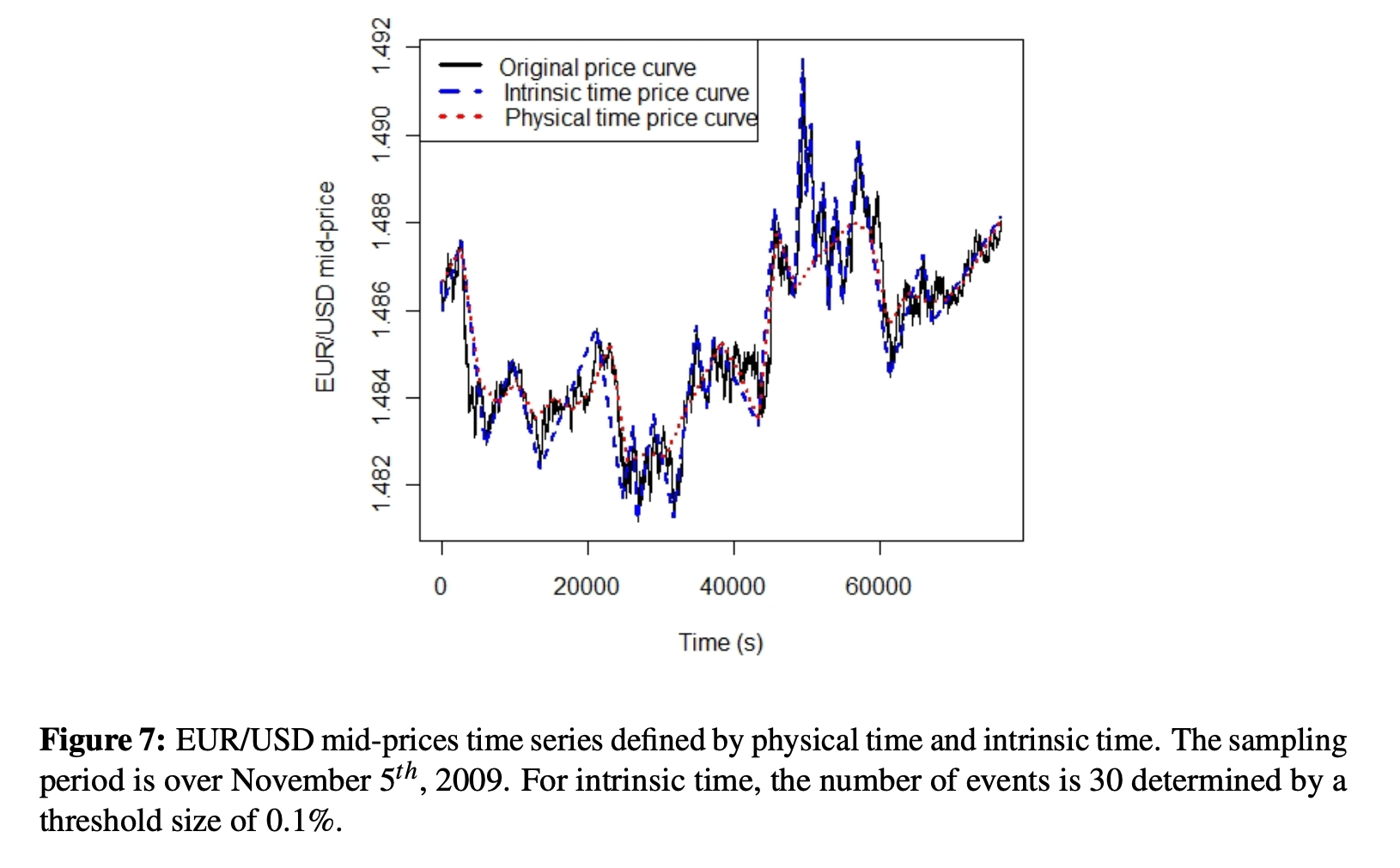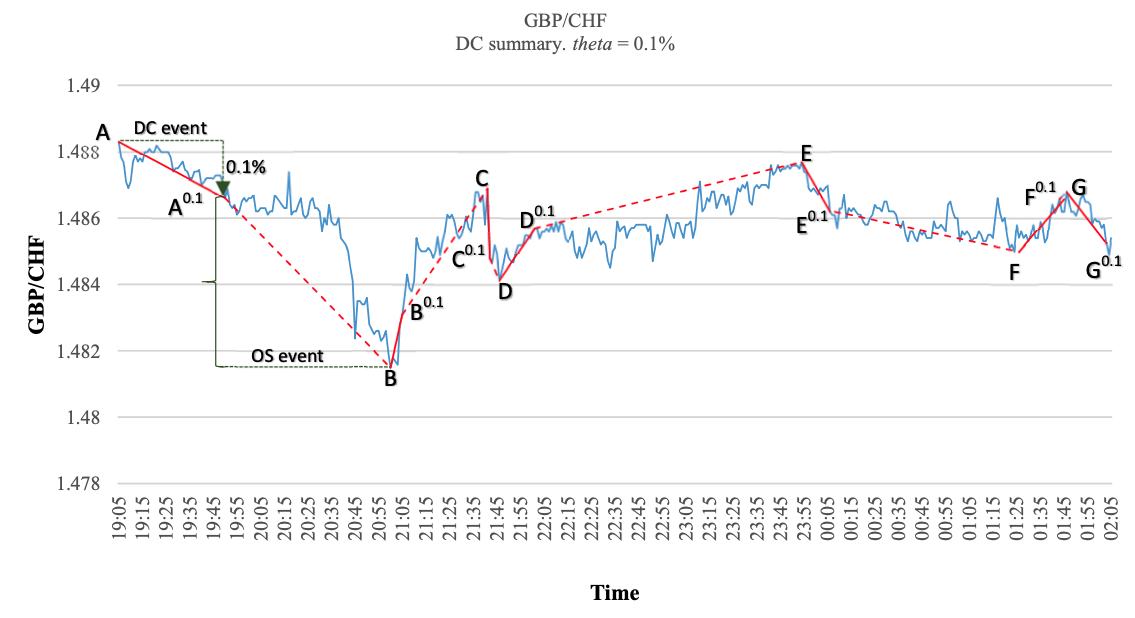# Directional changes in Python

hyangki0119·2022년 4월 19일
0# Intrinsic time and direciontal changes

## Physical time vs. Intrinsic time

• Physical time
• point-based
• daily, hourly,...
• Traditional approachs to observe price movements in financial time series are based on physical time changes
• It has become difficult and challenging to obeserve using this. Why?
• Significant patterns of the trading activity are ignored when using physical time
• Intrinsic time
• event-based
• What is event?
• an event is characterized by a fixed threshold $\lambda$
• is defined as the absolute price change between two local extremal values exceeding a given threshold $\lambda$
• Directional changes are used to define event
• Physical time vs. Intrinsic time
Physical timeIntrinsic time
point-basedevent-based
homogeneousinhomogeneous
time scales equally spacedtime triggers only at periodic events
• See Figure below:
• The lines in the graph summarize the price movements using intrinsic and physical time
• Significant events of trading activity are ignored when we consider changes over time based on fixed time intervals# Directional-change : definition

## Terminology### Extreme point / DCC point

• If the inequality just above holds,
TermDescriptionExample in figure
Extreme pointthe time at $P_{EXT}$points $A$, $B$, $C$, $D$, $E$, $F$, $G$
DCC pointthe time at $P_{c}$points $A^{0.1}$, $B^{0.1}$, $C^{0.1}$, $D^{0.1}$, $E^{0.1}$, $F^{0.1}$, $G^{0.1}$
• Note that whilst an extreme point is the end of the one trend, it is also the start point of the next trend
• An extreme point is only recognized in hindsight; precisely at the DCC point

### DC event / OS event

• DC means "Directional Change" and OS does "Overshoot"
TermDescriptionExample in figure
DC event-starts with an extreme point
-ends with a DCC point
intervals $[A,A^{0.1}]$, $[B,B^{0.1}]$, $\dots$, $[G,G^{0.1}]$
DCC point-starts at the DCC point
-ends at the next extreme point
ntervals $[A^{0.1},B]$, $[B^{0.1},C]$, $\dots$, $[E^{0.1},F]$
• Note that for a given time series and a predetermined threshold, the DC summary is unique

## Definition of DC

• A directional-change(DC) event can take one of the two forms
• a downturn event
• an upturn event
• Note that a downturn event occurs in upward run and an upturn event occurs in downward run
downward runupward run
A period between a downturn event and the next upturn eventA period between a upturn event and the next downturn event
The last low price $p_{L}$ is continuosly updated to the minimum of the current market price $p(t)$ and the last low price $p_{L}$The last high price $p_{L}$ is continuosly updated to the maximum of the current market price $p(t)$ and the last high price $p_{H}$
An $upturn$ event is an event when the absolute price change between the current market price $p(t)$ and the last low price $P_{L}$ is higher than a given threshold $\Delta x_{DC}$, i.e., $p(t)\ge p_{L}(1+\Delta x_{DC})$

If this holds, the point which the price last troughed ($P_{L})$ is the starting point of a upturn event
An $downturn$ event is an event when the absolute price change between the current market price $p(t)$ and the last high price $P_{H}$ is lower than a given threshold $\Delta x_{DC}$, i.e., $p(t)\le p_{H}(1-\Delta x_{DC})$

If this holds, the point which the price last peaked ($P_{H})$ is the starting point of a downturn event

# Implementation in Python

def myDC(data, d=0.2):
'''
- Aloud, M., Tsang, E., Olsen, R. & Dupuis, A. (2012). A Directional-Change Event Approach for Studying Financial Time Series. Economics, 6(1), 20120036.
- data : a list or array-like time series object
- d : theta value, which is a threshold to decide upturn/downturn event
'''

p = pd.DataFrame({
"Price": data
})
p["Event"] = ''
run = "upward" # initial run
ph = p['Price'] # highest price
pl = ph # lowest price
pl_i = ph_i = 0

for t in range(0, len(p)):
pt = p["Price"][t]
if run == "downward":
if pt < pl:
pl = pt
pl_i = t
if pt >= pl * (1 + d):
p.at[pl_i, 'Event'] = "start upturn event"
run = "upward"
ph = pt
ph_i = t
# print(">> {} - Upward! : {}%, value {}".format(pl_i, round((pt - pl)/pl, 2), round(pt - pl,2)))
elif run == "upward":
if pt > ph:
ph = pt
ph_i = t
if pt <= ph * (1 - d):
p.at[ph_i, 'Event'] = "start downturn event"
run = "downward"
pl = pt
pl_i = t
# print(">> {} - Downward! : {}%, value {}".format(ph_i, round((ph - pt)/ph, 2), round(ph - pt,2)))
return p